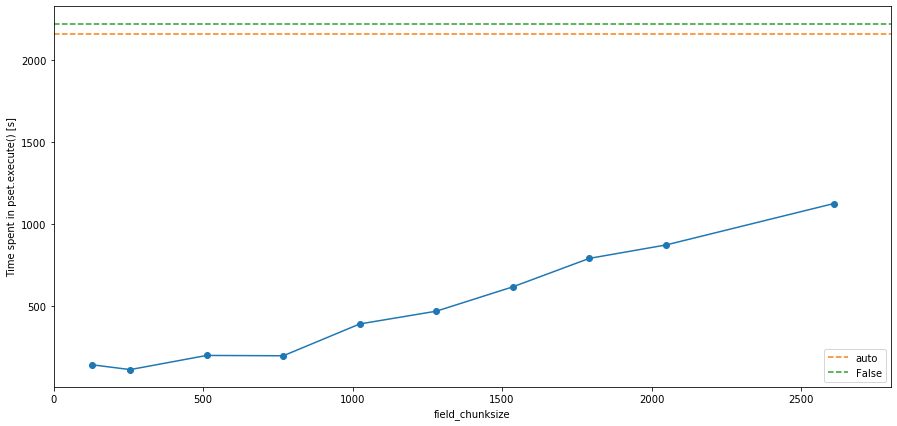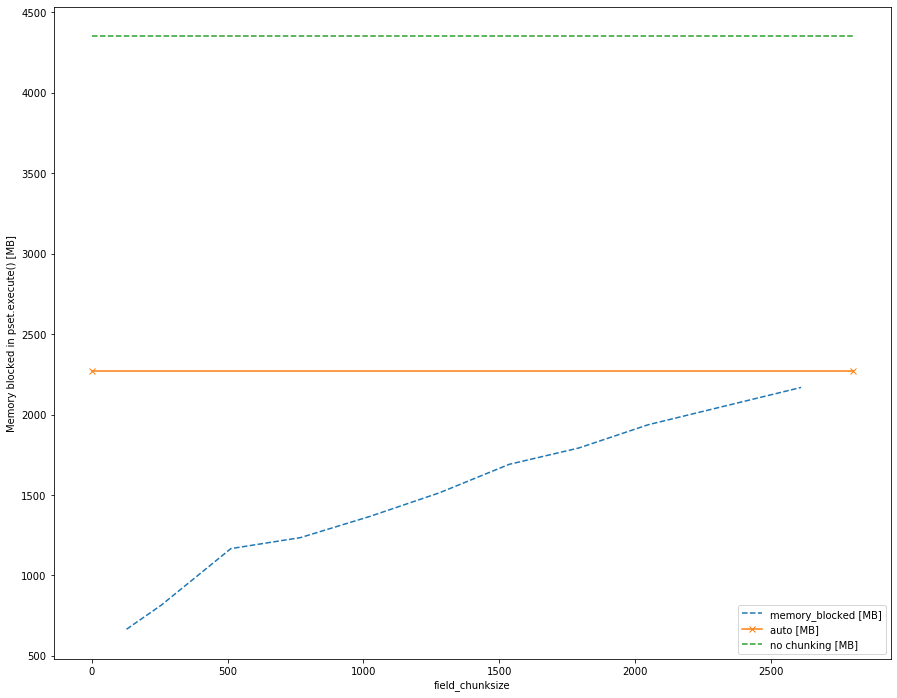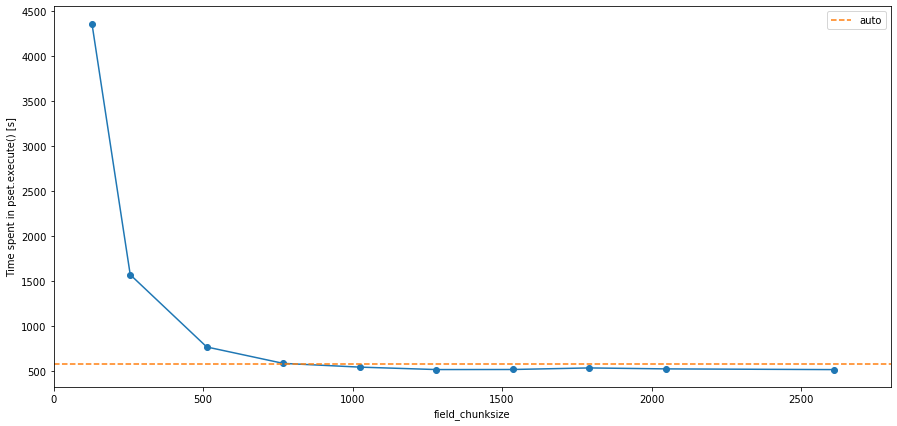## Tutorial on Parallel Parcels with MPI and dask field-chunking¶

Parcels can be run in Parallel with MPI. To do this, first follow the installation instructions at http://oceanparcels.org/#parallel_install.

### Splitting the ParticleSet with MPI¶

Once you have installed a parallel version of Parcels, you can simply run your script with

mpirun -np <np> python <yourscript.py>

Where <np> is the number of processors you want to use

Parcels will then split the ParticleSet into <np> smaller ParticleSets, based on a sklearn.cluster.KMeans clustering. Each of those smaller ParticleSets will be executed by one of the <np> MPI processors.

Note that in principle this means that all MPI processors need access to the full FieldSet, which can be Gigabytes in size for large global datasets. Therefore, efficient parallelisation only works if at the same time we also chunk the FieldSet into smaller domains

### Chunking the FieldSet with dask¶

The basic idea of field-chunking in Parcels is that we use the dask library to load only these regions of the Field that are occupied by Particles. The advantage is that if different MPI procesors take care of Particles in different parts of the domain, each only needs to load a small section of the full FieldSet (although note that this load-balancing functionality is still in development). Furthermore, the field-chunking in principle makes the indices keyword superfluous, as Parcels will determine which part of the domain to load itself.

The default behaviour is for dask to control the chunking, via a call to da.from_array(data, chunks='auto'). The chunksizes are then determined by the layout of the NetCDF files.

However, in tests we have experienced that this may not necessarily be the most efficient chunking. Therefore, Parcels provides control over the chunksize via the chunksize keyword in Field creation, which requires a dictionary that sets the typical size of chunks for each dimension.

It is strongly encouraged to explore what the best value for chunksize is for your experiment, which will depend on the FieldSet, the ParticleSet and the type of simulation (2D versus 3D). As a guidance, we have found that chunksizes in the zonal and meridional direction of approximately around 128 to 512 are typically most effective. The binning relates to the size of the model and its data size, so power-of-two values are advantageous but not required.

The notebook below shows an example script to explore the scaling of the time taken for pset.execute() as a function of zonal and meridional chunksize for a dataset from the CMEMS portal.

In :
%pylab inline
from parcels import FieldSet, ParticleSet, JITParticle, AdvectionRK4
from datetime import timedelta as delta
import numpy as np
from glob import glob
import os
import time
import psutil
import matplotlib.pyplot as plt

def set_cmems_fieldset(cs):
files = sorted(glob(data_dir+"mercatorglorys12v1_gl12_mean_201607*.nc"))
variables = {'U': 'uo', 'V': 'vo'}
dimensions = {'lon': 'longitude', 'lat': 'latitude', 'time': 'time'}

if cs not in ['auto', False]:
cs = {'time': ('time', 1), 'lat': ('latitude', cs), 'lon': ('longitude', cs)}
return FieldSet.from_netcdf(files, variables, dimensions, chunksize=cs)

func_time = []
mem_used_GB = []
chunksize = [128, 256, 512, 768, 1024, 1280, 1536, 1792, 2048, 2610, 'auto', False]
for cs in chunksize:

fieldset = set_cmems_fieldset(cs)
pset = ParticleSet(fieldset=fieldset, pclass=JITParticle, lon=, lat=, repeatdt=delta(hours=1))

tic = time.time()
func_time.append(time.time()-tic)
process = psutil.Process(os.getpid())
mem_used_GB.append(mem_B_used / (1024 * 1024))

Populating the interactive namespace from numpy and matplotlib

In :
fig, ax = plt.subplots(1, 1, figsize=(15, 7))

ax.plot(chunksize[:-2], func_time[:-2], 'o-')
ax.plot([0, 2800], [func_time[-2], func_time[-2]], '--', label=chunksize[-2])
ax.plot([0, 2800], [func_time[-1], func_time[-1]], '--', label=chunksize[-1])
plt.xlim([0, 2800])
plt.legend()
ax.set_xlabel('chunksize')
ax.set_ylabel('Time spent in pset.execute() [s]')
plt.show()The plot above shows that in this case, chunksize='auto' and chunksize=False (the two dashed lines) are roughly the same speed, but that the fastest run is for chunksize=(1, 256, 256). But note that the actual thresholds and numbers depend on the FieldSet used and the specifics of your experiment.

Furthermore, one of the major advantages of field chunking is the efficient utilization of memory. This permits the distribution of the particle advection to many cores, as otherwise the processing unit (e.g. a CPU core; a node in a cluster) would exhaust the memory rapidly. This is shown in the following plot of the memory behaviour.

In :
fig, ax = plt.subplots(1, 1, figsize=(15, 12))
ax.plot(chunksize[:-2], mem_used_GB[:-2], '--', label="memory_blocked [MB]")
ax.plot([0, 2800], [mem_used_GB[-2], mem_used_GB[-2]], 'x-', label="auto [MB]")
ax.plot([0, 2800], [mem_used_GB[-1], mem_used_GB[-1]], '--', label="no chunking [MB]")
plt.legend()
ax.set_xlabel('chunksize')
ax.set_ylabel('Memory blocked in pset.execute() [MB]')
plt.show()It can clearly be seen that with chunksize=False (green line), all Field data are loaded in full directly into memory, which can lead to MPI-run simulations being aborted for memory reasons. Furthermore, one can see that even the automatic method is not optimal, and optimizing the chunksize for a specific hydrodynamic dataset can make a large difference to the memory used.

It may - depending on your simulation goal - be necessary to tweak the chunksize to leave more memory space for additional particles that are being simulated. Since particles and fields share the same memory space, lower memory utilisation by the FieldSet means more memory available for a larger ParticleSet.

Also note that the above example is for a 2D application. For 3D applications, the chunksize=False will almost always be slower than chunksize='auto' or any dictionary, and is likely to run into insufficient memory issues, raising a MemoryError. The plot below shows the same analysis as above, but this time for a set of simulations using the full 3D CMEMS code. In this case, the chunksize='auto' is about two orders of magnitude faster than running without chunking, and about 7.5 times faster than with minimal chunk capacity (i.e. chunksize=(1, 128, 128)).

Choosing too small chunksizes can make the code slower, again highlighting that it is wise to explore which chunksize is best for your experiment before you perform it.

In :
from parcels import AdvectionRK4_3D
def set_cmems_fieldset_3D(cs):
files = sorted(glob(data_dir+"mercatorglorys12v1_gl12_mean_201607*.nc"))
variables = {'U': 'uo', 'V': 'vo'}
dimensions = {'lon': 'longitude', 'lat': 'latitude', 'depth': 'depth', 'time': 'time'}

if cs not in ['auto', False]:
cs = {'time': ('time', 1), 'depth': {'depth', 1}, 'lat': ('latitude', cs), 'lon': ('longitude', cs)}
return FieldSet.from_netcdf(files, variables, dimensions, chunksize=cs)

chunksize_3D = [128, 256, 512, 768, 1024, 1280, 1536, 1792, 2048, 2610, 'auto', False]
func_time3D = []
for cs in chunksize_3D:

fieldset = set_cmems_fieldset_3D(cs)
pset = ParticleSet(fieldset=fieldset, pclass=JITParticle, lon=, lat=, repeatdt=delta(hours=1))

tic = time.time()
func_time3D.append(time.time()-tic)

Computed 3D field advection.

In :
fig, ax = plt.subplots(1, 1, figsize=(15, 7))

ax.plot(chunksize[:-2], func_time3D[:-2], 'o-')
ax.plot([0, 2800], [func_time3D[-2], func_time3D[-2]], '--', label=chunksize_3D[-2])
plt.xlim([0, 2800])
plt.legend()
ax.set_xlabel('chunksize')
ax.set_ylabel('Time spent in pset.execute() [s]')
plt.show()The current implementation of MPI parallelisations in Parcels is still fairly rudimentary. In particular, we will continue to develop the load-balancing of the ParticleSet.
With load-balancing we mean that Particles that are close together are ideally on the same MPI processor. Practically, it means that we need to take care how Particles are spread over chunks and processors. See for example the two figures below:Example of load-balancing for Particles. The domain is chunked along the thick lines, and the orange and blue particles are on separate MPI processors. Before load-balancing (left panel), two chuncks in the centre of the domain have both orange and blue particles. After the load-balancing (right panel), the Particles are redistributed over the processors so that the number of chunks and particles per processor is optimised.
The difficulty is that since we don't know how the ParticleSet will disperse over time, we need to do this load-balancing 'on the fly'. If you to contribute to the optimisation of the load-balancing, please leave a message on github!